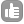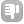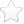0Iam bit stuck with % z gradient calculation used in Bruker pulse programs. In some instruments (Varian) they use as short as 200us for some gradient pulses, but in Bruker, they fix the length of all the gradient pulses as constant (say 500 us) and vary the gradient strength accordingly. Hence, is there any easy way to convert a gradient pulse of say 17 G/cm applied for 0.5 ms to 'x' G/cm for 1ms.(where 'x' is the new gradient strength for 1ms). Once this conversion is completed,the next step would be to calculate the gradient % which i think is the ratio of ('x' (G/cm)*100)/Maxgradamplitude, where Maxgradamplitude is the maximum G/cm specified for a spectrometer (e.g. 70 G/cm for an 800 MHz). (I read from Dr.James Keeler notes that the sum of the phase of the coherence before and after the application of a pair of z gradient pulses should be zero, for an appropriate coherence to be selected. The book also suggests an handy formulae (s1*Bg1t_1)/(s_2Bg2*t2) = -p1/p2; where s1,s2 are shape factors of gradient pulses used for coherence selection. Bg1,Bg2 are the gradient field strength of first and second gradient pulse, t1,t2 are the time period for each applied pulse and p1 and p2 are the coherence before and after the gradient pulses, respectively. But this formulae requires the complete coherence transfer pathway to be elucidated.On the other hand, the published sequence would have considered all these details while providing the strength and delays of gradient pulses. So is it possible to take advantage of the already existing information and make the conversion for desired delays in a simplied way.) asked Jun 12 '12 at 12:28Jana 311What you need to know is that for coherence selection it is the ratio of the integrals of the gradient pulses that is important, not the absolute intensity. The integral of the gradient is the intensity x length x integral of gradient shape. Since on any given spectrometer all the gradients usually have the same shape (rectangular on Varian, and historically half-Sine on Bruker), then the integral of the shape drops out, and you can just calculate the product of the intensity and length. For example in an echo-antiecho 1H-13C HSQC experiment, we use gradients in the ratio 80:20.1 (= ratio of magnetogyric ratio of proton and carbon). We can acheive this by having gradient pulses of equal length, and intensity 80% and 20.1%, or we could use gradients of the same intensity and length e.g. 800 and 201 us, or any equivalent combination. So for example if the Varian sequence has gradients of 0.2, 0.5 and 0.8 ms all at the same power, on the Bruker system you can just use a gradient pulse of 1ms at power levels of 20%, 50% and 80% respectively. The absolute intensity of the gradient pulses affects the extent that the unwanted coherences are suppressed, but unless you are suppressing protonated solvent signals this is not usually a problem. Typically we use 1ms pulses, with intensities in the 10-90% range. You should not use gradients above 95%, as the amplifier may be nonlinear in this range. answered Jun 15 '12 at 08:14Pete Gierth 401 Thanks Pete Gierth for the detailed clarification! - Jana (Jun 15 '12 at 09:29)